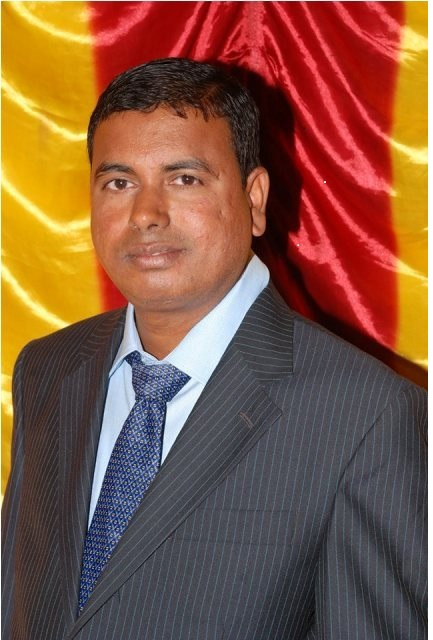Differential GeometryTeacher

Dr Tumbanath SamantaraCategory

Course Attendees

Still no participant

Still no reviews

Code(Credit) : CUTM1537(3-0-1)

Course Objectives

• To study about different geometrical figure and their representation in mathematical equation
• To study about  notations and operations of  Tensor.

Learning Outcomes

• Upon successful completion of this course,students will be able to

•  write equation of normal , binormal and  tangent to a curve.
•  able to understand tensorial expressions .

Course Syllabus

Module-I

Introduction to Differential Geometry,Introduction to Differential Geometry,Osculating plane and Rectifying Plane

Project 1:.finding the direction of tangent , normal and binormal at any point of curve

Module-II

Curvatures of a curve at a point,Torsion of a curve at a point,Expression of Curvature and Torsion in terms of arc length parameter,Expression of Curvature and Torsion in terms of arbitrary parameter

Project 2: Compute the Curvature of an ellipse.

Module-III

Spherical Indicatrix, Evolutes , Involutes

Project 3: Determine the evolutes of the given curve.

Module-IV

Betrand Curve,Osculating Spheres , Osculating circles.

Project-4 : Show that the tangent to the locus of osculating sphere passes through  the centre of the Oscilating Circle.

Module-V

Surface: Tangent planes and Normals,The two fundamental forms

Project 5: Find the normal to a given surface

Module-VI

Tensor : Definitions and explanations,Vector Space,Free systems, Basis and Dimension,Suffix Conventions,Transformation law for change of Basis Vectors and Components ,Dual Spaces

Module-VII

Transformation law for change of Basis in dual Space,Isomorphism,Tensor Product of Vector Spaces, Real Valued Bilinear Functions,Special Tensors

Project-6: Show that the velocity of a fluid at any point is component of a contravariant vector

BOOK PRESCRIBED

1. A text book of vector calculus-Shanti Narayana and J.N.Kapoor

Chapters: II and III

2. An Introduction to Differential Geometry by T.G. Willmore -Oxford University Press (1983)

Chapters : V

BOOK FOR REFERENCE

1.Differential Geometry-P.P.Gupta,G.S.Malik, S.K.Pundir

2.Tensor Analysis- Edward Nelson( Princeton University Press & University of Tokyo Press),1967

3.Introduction to Tensor Analysis and the Calculus of Moving Surfaces-Pavel Grinfeld, Springer

https://www.powershow.com/view1/237cec-ZDc1Z/Differential_Geometry_with_Applications_powerpoint_ppt_presentation

Session 1

Introduction to Differential Geometry

Osculating plane

Rectifying Plane

Session 5:

Serret-Frenet Formulae

Session 6&7

Project- 1: Finding the direction of tangent , normal and binormal at any point of    the curve

Session 8:

Curvatures of a curve at a point

Session 9:

Torsion of a curve at a point

Session 10:

Expression of Curvature and Torsion in terms of arc length parameter

Session 11:

Expression of Curvature and Torsion in terms of arbitrary parameter

Session 12&13:

Project-2: Compute the Curvature of an ellipse.

Session 14:

Spherical Indicatrix,

Evolutes

Involutes

Session 17&18:

Project 3: Determine the evolutes of the given curve.

Betrand Curve

Session 20:

Osculating Spheres

Session 21:

Osculating circles

Session 22&23:

Project-4 : Show that the tangent to the locus of osculating sphere passes through  the centre of the Oscilating Circle.

Session 24

Surface: Tangent planes

Surface: Normals

Session 26

The First  Fundamental Forms

Session 27

The Second  Fundamental Forms

Session 28&29:

Project-5 :Find the normal to a given surface

Session 30 :

Tensor : Definitions and explanations

Vector Spaces

Session 32:

Free systems, Basis and Dimension

Session 33:

Suffix Conventions

Session 34:

Transformation law for change of Basis Vectors and Components

Dual Spaces

Session 36:

Transformation law for change of Basis in dual Space

Session 37:

Tensor Product of Vector Spaces.

Session 38:

Real Valued Bilinear Functions

Session 39:

Components of Tensor

Special  Tensors

Session- 41&42

Project-6: Show that the velocity of a fluid at any point is component of a contravariant vector

Case Studies